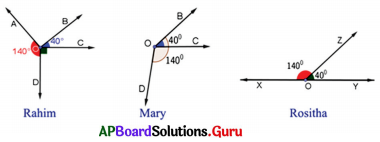SCERT AP 7th Class Maths Solutions Pdf Chapter 4 Lines and Angles Ex 4.2 Textbook Exercise Questions and Answers.

## AP State Syllabus 7th Class Maths Solutions 4th Lesson Lines and Angles Exercise 4.2

Question 1.
Observe the given figure and write any 2 linear pairs of angles.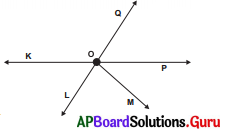Linear pair of angles :
∠KOQ, ∠QOP
∠KOQ, ∠KOL
∠KOL, ∠LOP
∠QOP, ∠POL
∠KOM, ∠MOP
∠QOM, ∠MOL

Question 2.
Draw a pair of adjacent angles which are complementary to each other.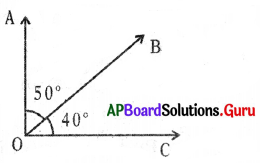In the figure ∠AOB, ∠BOC are adjacent angles and their sum is complementary.
∴ ∠AOB + ∠BOC = 50° + 40° = 90°

Question 3.
Draw a pair of adjacent angles which are supplementary to each other.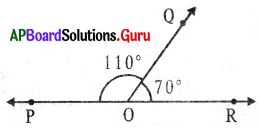In the figure ∠POQ, ∠OOR are linear pair and adjacent angles and their sum is supplementary.
∴ ∠POQ + ∠OOR = 110° + 70° = 180°

Question 4.
Give any two examples of adjacent angles in your surroundings.
Fan, Bullock cart wheel, Clock, etc.Question 5.
Observe the figure and write the adjacent angles.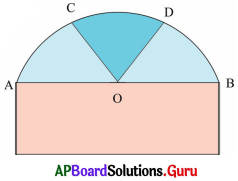In the given figure, adjacent angles are
∠AOC, ∠COB
∠AOC, ∠COD
∠COD, ∠DOB
∠AOD, ∠DOB

Question 6.
Is it possible for the following pair of angles to form a linear pair? If yes, draw them. If not, give reason.
(i) 120°, 60°
Yes.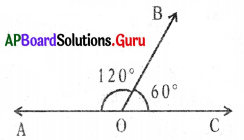120° + 60° = 180°
120°, 60° (Linear pair)

(ii) 98°, 102°
No, their sum is not equal to 180°
98° + 102° = 200° > 180°
So, they are not 1irear pair.

Question 7.
Draw the following angles as linear pair. Write the straight line and common arm.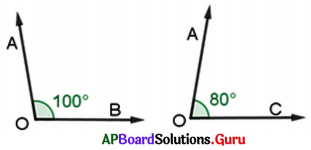∠BOA + ∠AOC = 180°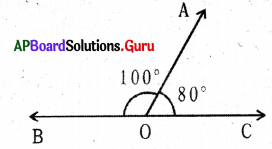∴ ∠BOA and ∠AOC are linear pair.
BC is a straight line and OA is an arm.

Question 8.
In the given figure, AB is a straight line. O is a point on AB . Find the value of x.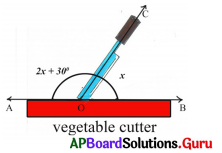In the given figure $$\overrightarrow{\mathrm{AB}}$$ is a straight line.
∠AOC + ∠COB = 180°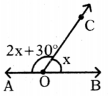⇒ 2x + 30° + x = 180°
⇒ 3x + 30°= 180°
⇒ 3x + 30° – 30°= 180°
⇒ 3x = 150°
⇒ $$\frac{3 x}{3}=\frac{150^{\circ}}{3}$$
⇒ x = 50Question 9.
Teacher asked students to check whether 40° and 140° form a linear pair or not by drawing angles. The students have drawn as follows. Who will get correct answer?